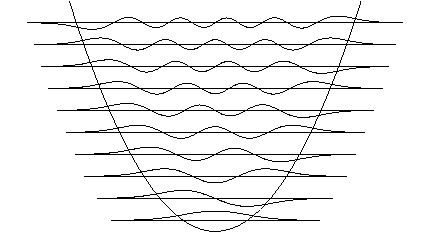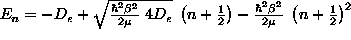# Solving the Schrödinger equation in one dimension

Here we give a simple Fortran code that calculates the eigenstates of the Schrödinger equation in one dimension, given a potential. The idea of the program is very simple: Potential and wavefunctions are discretized and the second derivative in the kinetic energy is approximated as a finite difference. Thus the Hamiltonian becomes a matrix, whose eigenvalues can be found using standard LAPACK routines. An explicit way of solving the eigenvalue problem would involve trial integrations of the Schroedinger equation and changing the trial energy until a state is found that has the proper boundary conditions. A very nice discussion of this procedure is given in the Feynman Lectures, Vol.3, Sec.16-6
To compile and run under Unix, enter: g77 qm1d.f && a.out
You can find the libarary routines also on GAMS: Search for module name dstevx

To familiarize yourself with the code, have a look at qm1d.f and try to understand its structure:

• choose units and specify potential
• set up Hamiltonian
• call library routine to find eigenvalues and eigenfunctions
• prepare plot fileHere are some suggestions of what you could do:

1. Run the code to get the ten lowest eigenstates for a particle in a box.
• Compare the numerical eigenenergies with the exact result.
• Change the width of the box (dx=width/dble(NPTS-1)). What happens?
• Why do the wavefunctions vanish at the boundaries? Look at the finite difference expression of the second derivative at the boundaries. What assumption has been made?
2. Change the potential to a harmonic oscillator centered on the mesh.
• How should the spring constant be choosen? We want the nst lowest eigenstates, and the mesh should be large enough that the eigenfunctions have essentially decayed to zero on the boundaries. Remember the exact result v(x)=(a*x)^2 ==> ee(n)=(2*n-1)*a Thus for v(x) centered in the box, choose a such that (a*width/2)^2 >> (2*nst-1)*a
example:
      dx=10d0/dble(NPTS-1)
write(*,'("harmonic oscillator")')
nst=10
do i=1,NPTS
v(i)=4d0*(dble(i-1)-dble((NPTS-1)/2))**2*dx**2
enddo

• Compare with exact solution. What happens when you
• increase/decrease the number of mesh points (try NPTS=51, 101, 201, 401)
• increase/decrease a on a fixed mesh (try a=2.0, 3.0, 4.0, 5.0 6.0)
3. To improve the numerical accuracy, you could implement the Numerov method. But attention! The program as it stands assumes a symmetric Hamiltonian. So when implementing the Numerov method, you also have to use a more general diagonalization routine.
4. For potentials with inversion symmetry the wavefunctions have parity. For example: The ground state of the harmonic oscillator is symmetric with respect to the center of the potential (even parity), while the first excited state is antisymmetric (odd parity). It would be thus enough to calculate the wavefunction on only one half of the potential.
• run the code for a harmonic potential centered on the left boundary of the mesh. What states do you find?
• States with even parity do not vanish at the center of the potential, instead they have a vanishing first derivative. Change the boundary conditions in the kinetic energy term such that you obtain the even parity states (assume phi(-dx)=phi(dx)). Unfortunately this makes the Hamiltonian matrix non-symmetric, so we cannot use the simple diagonalization routine (which can only handle tridiagonal symmetric matrices) here. A similar problem appears for periodic boundary conditions. Then the Hamiltonian is no longer tridiagonal.

In these cases, you have to use a general diagonalization routine. Something like that can be very easily implemented in systems like Matlab, Octave, IDL, or PV-WAVE. A brief PV-WAVE code looks, e.g., like

n=100
a=0.0005
H=dblarr(n+1,n+1)
V=dblarr(n+1)
for i=0,n do begin
V(i)=a*double(i-n/2)^2
H(i,i)=2d0+V(i)
if(i gt 0) then H(i,i-1)=-1d0
if(i lt n) then H(i,i+1)=-1d0
endfor
ee=eig(H,vectors=ev,/symmetric)

de=ee(n-1)-ee(n)
plot, V, yrange=[0,ee(n-11)]
for i=n-10,n do begin
oplot, [1,n], [1,1]*ee(i)
oplot, ee(i)+1.5*de*ev(*,i)
endfor
end

5. Comparing with exactly known results is always an essential step when working with numerical methods. It allows you to (i) check if the code is correct and accurate and (ii) you make sure that you really understand what the code is doing (choice of units, influence of boundary conditions, etc.)

Now you are ready to go beyond exactly solvable cases

6. Try the anharmonic oscillator
• v(x)=a1*x+a2*x^2+a3*x^3. The shifted harmonic oscillator can be solved exactly; the x^3 term gives a good model for the anharmonicity of molecular vibrations (see, e.g., C.Cohen-Tannoudji, B.Diu, F.Laloe: Quantum Mechanics, Vol.2, Complement A.XI)

To study molecular vibrations you can also use the Morse potentialwhich, btw, is exactly solvable using supersymmetric quantum mechanics and has eigenenergiesFor a very brief introduction to supersymmetric quantum mechanics, see E. Koch: Superschalen in Metallclustern, Sec. 1.2
• v(x)=a2*x^2+a4*x^4, a4>0 For a2<0 you get a double well potential. Observe how, deep in the wells, you get pairs of states with very similar energy. What is the parity of the state with lower energy? (bonding/antibonding states).
7. Try a sinusoidal potential with 2, 3, 4, ... periods on the mesh (increase NPTS as required!) and observe groups of eigenstates. What would you get for a potential with very many periods on the mesh? (you can also try a finite Kramers-Kronig model)
8. More things to try: lateral potential for 2-dim electron gas; use wavefunctions to calculate matrix elements

back
Erik Koch